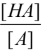# Problem: Which of the following statements regarding the Henderson-Hasselbalch (HH) equation are correct?I The HH equation is pH = pKa + log II The HH equation can be used whenever the concentrations of [HA] and [A-] are nonzero.III If [HA] equals 50-times [A-], the HH equation states that the pH will be 1.7 units higher than the pKa of HA.A. All statements are correctB. Only statements I and II are correctC. Only statements II and III are correctD. Only statement I is correctE. None of the statements is correct

###### FREE Expert Solution
94% (413 ratings)View Complete Written Solution
###### Problem Details

Which of the following statements regarding the Henderson-Hasselbalch (HH) equation are correct?
I The HH equation is pH = pKa + logII The HH equation can be used whenever the concentrations of [HA] and [A-] are nonzero.

III If [HA] equals 50-times [A-], the HH equation states that the pH will be 1.7 units higher than the pKa of HA.

A. All statements are correct

B. Only statements I and II are correct

C. Only statements II and III are correct

D. Only statement I is correct

E. None of the statements is correct